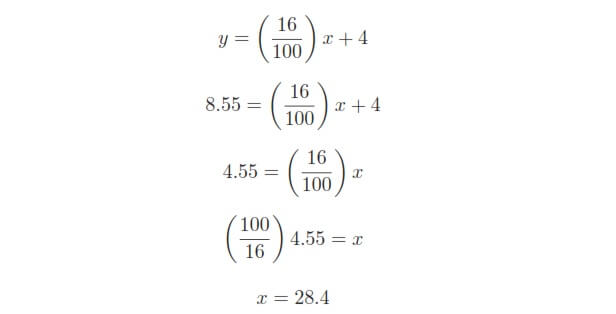# Calculate Control Valve Stem Position

## Calculate Control Valve Stem PositionWe are solving this problem using standard 4-20mA conversion Formula. Click here for formula

To solve for percentage of stem travel (x) at 8.55 milliamps of signal current (y), we may use the linear equation developed previously to predict  current in milliamps (y) from signal value in percent (x):Therefore, we should expect the valve to be 28.4% open at an applied MV signal of 8.55 milliamps.

We can also solve the problem using direct formula. Click here for formula

Be the first to get exclusive content straight to your email.
We promise not to spam you. You can unsubscribe at any time.

### 4 thoughts on “Calculate Control Valve Stem Position”

1.Very good

2.3.4.